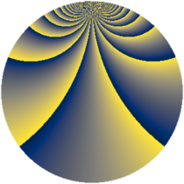# Properties

 Label 1148.2.cfLevel $1148$ Weight $2$ Character orbit 1148.cf Rep. character $\chi_{1148}(121,\cdot)$ Character field $\Q(\zeta_{60})$ Dimension $448$ Sturm bound $336$

# Learn more about

## Defining parameters

 Level: $$N$$ $$=$$ $$1148 = 2^{2} \cdot 7 \cdot 41$$ Weight: $$k$$ $$=$$ $$2$$ Character orbit: $$[\chi]$$ $$=$$ 1148.cf (of order $$60$$ and degree $$16$$) Character conductor: $$\operatorname{cond}(\chi)$$ $$=$$ $$287$$ Character field: $$\Q(\zeta_{60})$$ Sturm bound: $$336$$

## Dimensions

The following table gives the dimensions of various subspaces of $$M_{2}(1148, [\chi])$$.

Total New Old
Modular forms 2784 448 2336
Cusp forms 2592 448 2144
Eisenstein series 192 0 192

## Trace form

 $$448q + O(q^{10})$$ $$448q + 4q^{11} - 12q^{15} + 6q^{17} - 4q^{19} - 56q^{25} - 12q^{27} + 32q^{29} - 8q^{31} + 110q^{33} + 24q^{35} - 42q^{37} - 44q^{41} + 40q^{43} + 40q^{45} + 52q^{47} + 20q^{49} - 42q^{51} - 26q^{53} - 4q^{55} - 40q^{57} - 12q^{59} - 80q^{61} + 34q^{63} + 12q^{65} + 20q^{67} - 12q^{69} + 4q^{71} + 90q^{75} + 20q^{77} + 18q^{79} + 216q^{81} - 208q^{83} - 72q^{85} + 22q^{89} + 100q^{93} + 8q^{95} - 52q^{97} - 80q^{99} + O(q^{100})$$

## Decomposition of $$S_{2}^{\mathrm{new}}(1148, [\chi])$$ into newform subspaces

The newforms in this space have not yet been added to the LMFDB.

## Decomposition of $$S_{2}^{\mathrm{old}}(1148, [\chi])$$ into lower level spaces

$$S_{2}^{\mathrm{old}}(1148, [\chi]) \cong$$ $$S_{2}^{\mathrm{new}}(287, [\chi])$$$$^{\oplus 3}$$$$\oplus$$$$S_{2}^{\mathrm{new}}(574, [\chi])$$$$^{\oplus 2}$$